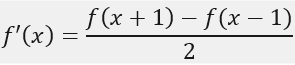# 2. 具体实例#include <iostream>
#include <opencv2\opencv.hpp>

using namespace cv;
using namespace std;

int main()
{
//从文件中读取成灰度图像
const char* imagename = "D:\\Data\\imgDemo\\lena.jpg";
if (img.empty())
{
fprintf(stderr, "Can not load image %s\n", imagename);
return -1;
}

//OpenCV函数进行一维卷积（梯度图）
Mat xKernel = (Mat_<double>(1, 3) << -1, 0, 1);			//卷积算子
Mat Ix;
filter2D(img, Ix, -1, xKernel);

//自建算法进行一维卷积（梯度图）
Mat Ixx;
Ixx.create(img.cols, img.rows, CV_8UC1);
double xk = { -1, 0, 1 };			//卷积算子
for (int i = 0; i < img.rows; ++i)
{
for (int j = 0; j < img.cols; ++j)
{
//img.at<uchar>(i, j) = 255;
uchar b = { 0 };
b = (j == 0 ? 0 : img.at<uchar>(i, j - 1));
b = img.at<uchar>(i, j);
b = (j == img.cols - 1 ? 0 : img.at<uchar>(i, j + 1));

double value = xk * b + xk * b + xk * b;
value = (std::min)(std::max(value, 0.0), 255.0);
Ixx.at<uchar>(i, j) = (uchar)value;
}
}

//比较两者的结果
Mat c;
compare(Ix, Ixx, c, CMP_EQ);

//显示图像
imshow("原始", img);
imshow("梯度图(CV)", Ix);
imshow("梯度图(MY)", Ixx);
imshow("比较结果", c);

waitKey();
return 0;
}# 3. 图像梯度图# 4. 参考资料

1.图像梯度的基本原理
2.图像梯度计算

posted @ 2019-03-23 00:13  charlee44  阅读(2706)  评论(0编辑  收藏